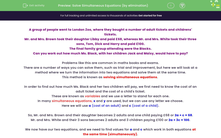# Solve Simultaneous Equations (by elimination)

In this worksheet, students will learn how to solve simple simultaneous equations using the elimination method. They will also solve contextualised question based on simultaneous equations.Key stage:  KS 4

Year:  GCSE

GCSE Subjects:   Maths

GCSE Boards:   Pearson Edexcel, OCR, Eduqas, AQA,

Curriculum topic:   Algebra

Curriculum subtopic:   Solving Equations and Inequalities Algebraic Equations

Difficulty level:#### Worksheet Overview

A group of people went to London Zoo, where they bought a number of adult tickets and childrens' tickets.

Mr. and Mrs. Brown took their daughter Libby and paid £68, whereas Mr. and Mrs. White took their three sons, Tom, Dick and Harry and paid £100.

The final family group attending were the Blacks.

Can you work out how much Ms. Black, with her children Jack and Maisy, would have to pay?

Problems like this are common in maths books and exams.

There are a number of ways you can solve them, such as trial and improvement, but here we will look at a method where we turn the information into two equations and solve them at the same time.

This method is known as solving simultaneous equations

In order to find out how much Ms. Black and her two children will pay, we first need to know the cost of an adult ticket and the cost of a child's ticket.

These are known as variables and we use a letter to stand for each one.

In many simultaneous equations, x and y are used, but we can use any letter we choose.

Here we will use a (cost of an adult) and c (cost of a child).

So, Mr. and Mrs. Brown and their daughter becomes 2 adults and one child paying £68 or 2a + c = 68.

Mr. and Mrs. White and their 3 sons becomes 2 adults and 3 children paying £100 or 2a + 3c = 100.

We now have our two equations, and we need to find values for a and c which work in both equations at the same time (simultaneously).

If we line up the equations, one above the other with the one with the biggest values on top it will help:

2a + 3c = 100

2a + c  =  68

We can see that both equations have 2a in them, but the top one has 2c more than the bottom one and the cost is £32 more, so 2c = 32.

If 2 children cost £32 then one must cost £16, so c =16.

Knowing these two facts, we can know work out a.

If 2c + c = 68 and c = 16 then 2c + 16 = 68.

We can solve this equation easily by subtracting 16 from both sides giving 2a = 52, and dividing by 2 gives a = 26.

So an adult ticket costs £26.

Now we have our two ticket costs, it is a good idea to check that they work for the other family (the equation we have not yet used).

We had 2a + 3c = 100 and substituting a = 26 and c= 16 gives 2 x 26 + 3 x 16 = 52 + 48 = 100, which is correct.

Now we can find the cost for the Black family, which is one adult and 2 children:

26 + 2 x 16 = 58, so they would pay £58.

Now, let's look at another pair of simultaneous equations.

This time just the equations have been given without the real-life problem.

Solve  5x + y = 17 and 3x + y = 11

Let's number our equations (1) and (2) with the bigger values on top then subtract equation (2) from (1):

5x + y = 17   (1)

3x + y = 11   (2)

2x = 6    (1) - (2)

x = 3    (divide by 2)

Substitute x = 3 into (2) and solve the equation [you could use equation (1) if you prefer]:

3 x 3 + y = 11

9 + y = 11

y = 2

So x = 3 and y = 2

Let's check these values work with equation (1) [i.e. the equation we did not use to find our values]

5 x 3 + 2 = 17 (which is correct)

Let's look at one more problem to check you have it.

Solve  5x - y = 17 and 2x + y = 11

5x - y = 17  (1)

2x + y = 11  (2)

Notice that the y's have the same coefficients (number in front) and so this is the variable which will be eliminated when we combine the equations.

Because the signs in front of the equations are different (one positive and one negative) this time we need to add them:

7x = 28   (1) + (2)

x = 4   [divide by 7]

2 x 4 + y = 11   [substitute into (2)]

8 + y = 11

y = 3

Let's check this in (1):

5 x 4 - 3 = 17 [correct]

So x = 4 and y = 3.

TOP TIPS:

Remember the variable with equal coefficients will be eliminated when the equations are combined.

If the signs in front are the same, you subtract, but if they are different then you add.

Remember the catchphrase: Same Sign Subtract (SSS) to help you commit this rule to memory.

In this activity, we will find the values of variable by solving pairs of simultaneous equations.

You may want to have a pen and paper handy to record your working and so you can compare it to our maths teacher's method.

### What is EdPlace?

We're your National Curriculum aligned online education content provider helping each child succeed in English, maths and science from year 1 to GCSE. With an EdPlace account you’ll be able to track and measure progress, helping each child achieve their best. We build confidence and attainment by personalising each child’s learning at a level that suits them.

Get started